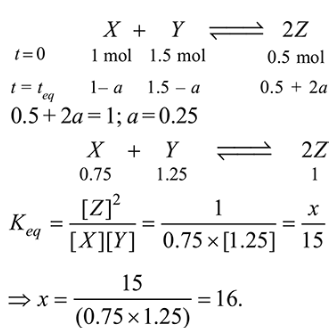# Solve the following`
Question:

For a reaction $\mathrm{X}+\mathrm{Y} \rightleftharpoons 2 \mathrm{Z}, 1.0 \mathrm{~mol}$ of $\mathrm{X}, 1.5 \mathrm{~mol}$ of $\mathrm{Y}$ and $0.5 \mathrm{~mol}$ of $Z$ were taken in a $1 \mathrm{~L}$ vessel and allowed to react. At equilibrium, the concentration of $\mathrm{Z}$ was $1.0 \mathrm{~mol} \mathrm{~L}^{-1}$. The equilibrium constant of the reaction is $\frac{x}{15}$. The value of $x$ is____________ .

Solution:

(16)